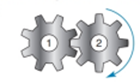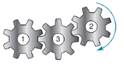Chapter 2.6, Problem 24EElementary Geometry for College St...

6th Edition
Daniel C. Alexander + 1 other
ISBN: 9781285195698

Solutions

Chapter
SectionElementary Geometry for College St...

6th Edition
Daniel C. Alexander + 1 other
ISBN: 9781285195698
Textbook Problem

In which direction (clockwise or counterclockwise) will gear   1 rotate if gear 2 rotates in the clockwise direction? a)b)To determine

a)

The direction in which will gear 1 rotate.

Explanation

Given:

The gear 2 rotates in clockwise direction.

The given figure is,

Figure (1)

Approach

To determine

b)

The direction in which will gear 1 rotate.

Still sussing out bartleby?

Check out a sample textbook solution.

See a sample solution

The Solution to Your Study Problems

Bartleby provides explanations to thousands of textbook problems written by our experts, many with advanced degrees!

Get Started

Find the maximum profit P (in dollars) given that 6(P 2500) 4(P + 2400)

Applied Calculus for the Managerial, Life, and Social Sciences: A Brief Approach

Evaluate the expression sin Exercises 116. 33

Finite Mathematics and Applied Calculus (MindTap Course List)

In problems 17-30, simplify each expression so that only positive exponents remain. 29.

Mathematical Applications for the Management, Life, and Social Sciences

What are the two requirements for a random sample?

Essentials of Statistics for The Behavioral Sciences (MindTap Course List)

Find y if xy = yx.

Single Variable Calculus: Early Transcendentals, Volume I

Evaluate each expression: 6+2(542)

Elementary Technical Mathematics

Sometimes, Always, or Never: If f(x) is continuous on [a, b], then abf(x) dx exists.

Study Guide for Stewart's Single Variable Calculus: Early Transcendentals, 8th

The equation of the line through (4, 10, 8) and (3, 5, 1) is:

Study Guide for Stewart's Multivariable Calculus, 8th